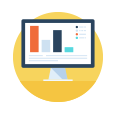# Key Stage 2 - KS2 Maths

. -->

### Year 3 Maths

Subtraction with borrowing, calculation sharing/division, units of time, bar charts and much more is included in this comprehensive course.

### Year 4 Maths

Topics include multiplication using extended algorithms, introduction to decimals, comparing & ordering fractions to bar charts & pictograms.

### Year 5 Maths

Year 5 introduces multiples and factors of whole numbers, reducing fractions to lowest equivalent form and rounding decimals amongst other topics.

### Year 6 Maths

Algebraic equations, rounding decimals, dividing and multiplying fractions and problems with length, mass and area are all introduced in year 6.Targeted maths & English online tutoring tailored to your country's curriculum..Easily and accurately monitor progress and support them in their learning..Very affordable and comprehensive when compare with real life tutors..

## KS2 Maths Explained